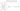← Go BackMarch 19, 20204 min read • IN tech

# Neural Net Problems - Exercise 1

I recently started reading Michael Nielson’s “Neural Networks and Deep Learning”. I have heard really good things about the website and Nielson’s teaching style. Here are my solutions to exercise 1.

## Sigmoid Neurons Simulating Perceptrons

### Part 1

#### Question

Suppose we take all the weights and biases in a network of perceptrons, and multiply them by a positive constant, $c>0$. Show that the behavior of the network doesn’t change.

#### Solution

The definition of a perceptron is:

$\begin{cases} 0 & \text{if } \sum_j w_j x_j \le \text{thresold}\\ 1 & \text{if } \sum_j w_j x_j > \text{thresold} \end{cases}$

or more nicely with the bias:

$\begin{cases} 0 & \text{if } b + \sum_j w_j x_j \le 0\\ 1 & \text{if } b + \sum_j w_j x_j > 0 \end{cases}$

Now if we multiple the bias and weight by the constant $c$ the result is:

\begin{aligned} \begin{cases} 0 & \text{if } cb + \sum_j c w_j x_j \le 0\\ 1 & \text{if } cb + \sum_j c w_j x_j > 0 \end{cases} &=\\ &= \begin{cases} 0 & \text{if } cb + c \sum_j w_j x_j \le 0\\ 1 & \text{if } cb + c \sum_j w_j x_j > 0 \end{cases}\\ &= \begin{cases} 0 & \text{if } c(b + \sum_j w_j x_j) \le 0\\ 1 & \text{if } c(b + \sum_j w_j x_j) > 0 \end{cases}\\ &= \begin{cases} 0 & \text{if } b + \sum_j w_j x_j \le 0\\ 1 & \text{if } b + \sum_j w_j x_j > 0 \end{cases} \end{aligned}

We end up back where we started from and thus multiplying by a constant $c$ (assuming $c > 0$) does not affect the behavior of the network.

### Part 2

#### Question

Suppose we have the same setup as the last problem - a network of perceptrons. Suppose also that the overall input to the network of perceptrons has been chosen. We won’t need the actual input value, we just need the input to have been fixed. Suppose the weights and biases are such that $w⋅x+b \ne 0$ for the input $x$ to any particular perceptron in the network. Now replace all the perceptrons in the network by sigmoid neurons, and multiply the weights and biases by a positive constant $c > 0$. Show that in the limit as $c \to \infty$ the behavior of this network of sigmoid neurons is the same as the network of perceptrons. How can this fail when $w \cdot x + b = 0$ for one of the perceptrons?

#### Solution

##### Sigmoid Neuron Defintion
$\frac{1}{1+\exp(-\sum_j w_j x_j -b)} = \frac{1}{1+\exp(-w \cdot x - b)}$
##### Assumptions

Assume that $x$ is a fixed unknown value where $w \cdot x + b \ne 0$.

Then the resulting network will remain unchanged (assuming $c > 0$) as $c \to \infty$.

By the way $\exp(x) \equiv e^x$

##### The Cases
$\frac{1}{1+\exp(c(-w \cdot x - b))}$

Let’s assume that $w \cdot x + b < 0$:

\begin{aligned} \lim_{c \to \infty} \frac{1}{1+\exp(c(-w \cdot x - b))} &= \\ &= \frac{1}{1+\exp( \lim_{c \to \infty} c(-w \cdot x - b))}\\ &= \frac{1}{1+\exp(\infty)}\\ &= \frac{1}{1+\infty}\\ &= 0 \end{aligned}

Let’s assume that $w \cdot x + b > 0$:

\begin{aligned} \lim_{c \to \infty} \frac{1}{1+\exp(c(-w \cdot x - b))} &= \\ &= \frac{1}{1+\exp( \lim_{c \to \infty} c(-w \cdot x - b))}\\ &= \frac{1}{1+\exp(-\infty)}\\ &= \frac{1}{1+0}\\ &= 1 \end{aligned}

This is exactly the behavior we would expect with a perceptron!

##### How Can This Fail?

If $w \cdot x + b = 0$ is true then:

\begin{aligned} \lim_{c \to \infty} \frac{1}{1+\exp(c(-w \cdot x - b))} &= \\ &= \frac{1}{1+\exp( \lim_{c \to \infty} c(0))}\\ &= \frac{1}{1+\exp(0)}\\ &= \frac{1}{1+1}\\ &= \frac{1}{2} \end{aligned}

$\lim_{c \to \infty} \frac{1}{1+\exp(c(-w \cdot x - b))} = \frac{1}{2} \ne 0$

This does not match the expected behavior of a perceptron.